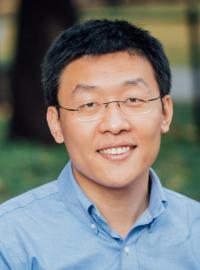Fully Discrete Second-order Linear Schemes for Hydrodynamic Phase Field Models of Binary Viscous Fluid Flows with Variable Densities
Speaker
A/Prof. Jia Zhao
Utah State University, USA
Abstract

In this talk, we present spatial-temporally second-order, energy stable numerical schemes for two classes of hydrodynamic phase field models of binary viscous fluid mixtures of different densities. One is quasi-incompressible while the other is incompressible. We introduce a novel invariant energy qudratization (IEQ) technique to arrive at fully discrete linear schemes, where in each time step only a linear system needs to be solved. These schemes are then proved to be unconditionally energy stable rigorously so that a large time step is plausible. Both spatial and temporal mesh refinements are conducted to illustrate the second order accuracy of the schemes. Several Numerical examples and conducted, and predictions by the two fluid mixture models are compared and discussed. As a conclusion, we believe the quasi-incompressible model is more reliable than the incompressible one.
This is a joint work with Yuezheng Gong (Nanjing University of Aeronautics and Astronautics, China), Xiaogang Yang (Wuhan Institute of Technology, China) and Qi Wang (Beijing Computational Science Research Center, China).